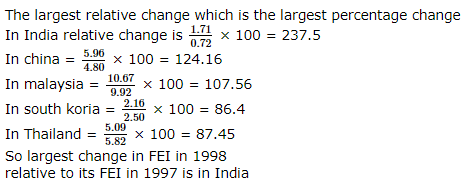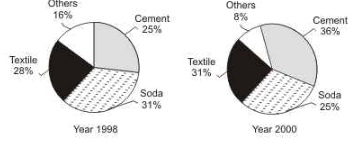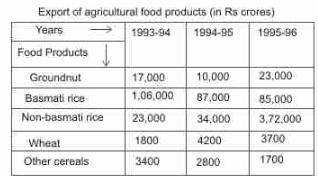Courses

# NABARD Manager Quant Aptitude Mock Test 8

## 20 Questions MCQ Test NABARD Manager - Mock Tests & Previous Year Papers | NABARD Manager Quant Aptitude Mock Test 8

Description
This mock test of NABARD Manager Quant Aptitude Mock Test 8 for Banking Exams helps you for every Banking Exams entrance exam. This contains 20 Multiple Choice Questions for Banking Exams NABARD Manager Quant Aptitude Mock Test 8 (mcq) to study with solutions a complete question bank. The solved questions answers in this NABARD Manager Quant Aptitude Mock Test 8 quiz give you a good mix of easy questions and tough questions. Banking Exams students definitely take this NABARD Manager Quant Aptitude Mock Test 8 exercise for a better result in the exam. You can find other NABARD Manager Quant Aptitude Mock Test 8 extra questions, long questions & short questions for Banking Exams on EduRev as well by searching above.
QUESTION: 1

Solution:
QUESTION: 2

### The average age of 34 boys in a class is 14 years. If the teacher's age is included the average age of the boys and the teacher becomes 15. What is the teacher's age?

Solution:

Teacher's age = 35 x 15 - 34 x14
= 525 - 476 = 49 years

QUESTION: 3

### The mean age of a combined group of men and women is 30 yrs. If the means of the ages of men and women are respectively 32 and 27,then the % of women in the group is

Solution:

Let the number of men and women in the group be m and n respectively.
Hence,
sum of the ages of the men in the group = mean age of men x number of men = 32m; and
sun of the ages of the women in the group = mean age of women x number of women = 27n
Also,
(Sum of ages of men + Sum of ages of women)/ Total people in group = Mean age of group
or (32m + 27n) (m + n) = 30
or 32m + 27n = 30m + 30n
or 2m = 3n
or m = 1.5n
Percentage of women in the group = 100n (m + n) = 100n 2.5n = 40%

QUESTION: 4
In 1 kg mixture of sand and iron, 20% is iron. How much sand should be added so that the proportion of iron becomes 10%?
Solution: In 1 kg mixture quantity of iron = 20 gm
Let x gm sand should be added, then 10% of (1000 + x) = 200
∴ x = 1000 gm = 1 kg
QUESTION: 5
If A:B=1:2,B:C=3:4 and C:D=5:6,Find D:C:B:A.
Solution:
QUESTION: 6
An article when sold for Rs 960 fetches 20% profit.What would be the percent profit/loss if 5 such articles are sold for Rs 825 each?
Solution:
QUESTION: 7
Choose the correct alternative that will continue the same pattern.
44, 41, 38, 35, 32, 29, 26,....?
Solution: This is a simple subtraction series, in which 3 is subtracted from each number to arrive at the next.
QUESTION: 8
Nitin bought 130 shirts at the rate of Rs 125 per shirt. The transport expenditure was Rs 350. He paid an octroi at the rate of Rs 1.50 per shirt and coolie charges were Rs 205. What should be the approximate selling price of one shirt, If he wants a profit of 10%?
Solution:
QUESTION: 9

Two squares are chosen at random on a chessboard. What is the probability that they have a side in common?

Solution:

The number of ways of choosing the first square is 64
The number of ways of choosing the second square is 63
There are a total of 64 x 63 = 4032 ways of choosing two squares
If the first square happens to be any of the four corner ones, the second square can be chosen in 2 ways
If the first square happens to be any of the 24 squares on the side of the chess board, the second square can be chosen in 3 ways
If the first square happens to be any of the 36 remaining squares, the second square can be chosen in 4 ways
Hence the desired number of combinations = (4x 2) + (24 x 3) + (36x 4) = 224
Therefore, the required probability = 224 /4032 = 1 18

QUESTION: 10
Rs 600 becomes Rs 720 in 4 years when the interst is simple. If the rate of intrest is increased by 2% then what will be total amount ?
Solution:
QUESTION: 11

A certain number of men can do a work in 40 days. If there were 8 men more, it could be finished in 10 days less. How many men were there initially?

Solution:
QUESTION: 12

The cost of painting the whole surface area of a cube at the rate of 13 paise per sq. cm is Rs. 343.98. Then the volume of the cube is :

Solution:
QUESTION: 13

In the following questions two equations numbered I and II are given. You have to solve both the equations and give answer.

I. x2 - 1200 = 244
II. y + 122 = 159

Solution:
QUESTION: 14

In the following questions two equations numbered I and II are given. You have to solve both the equations and give answer.

I. 14x - 25 = 59 - 7x
II. √y+222−√36=√81y+222−36=81

Solution:
QUESTION: 15

FEI for a country in a year, is the ratio (expressed as a percentage) of its foreign equity inflows to its GDP. The figure given displays the FEIs for select Asian countries for 1997 and 1998.

The country with the largest change in FEI in 1998, relative to its FEI in 1997, is :

Solution:QUESTION: 16

FEI for a country in a year, is the ratio (expressed as a percentage) of its foreign equity inflows to its GDP. The figure given displays the FEIs for select Asian countries for 1997 and 1998.

Based on the data provided, it can be concluded that :

Solution:

Absolute value is not given, hence nothing can be inferred.

QUESTION: 17

The percentage of revenue that comes from the four different prodcut segments for a diversiifed manufacturing company are shown in the following pie-charts. Fig-ures pertaining to the year 1998 and 2000 are given on the right hand side. The total sales in the year 1998 were Rs 120 crores. It was Rs 195 crores in 2000.Which product segment has registered the maximum percentage growth in revenue during the two year period?

Solution:

Cement has increased from 25% to 36%, which is the maximum, even if we don't take the increase in sales into account

QUESTION: 18

The percentage of revenue that comes from the four different prodcut segments for a diversiifed manufacturing company are shown in the following pie-charts. Fig-ures pertaining to the year 1998 and 2000 are given on the right hand side. The total sales in the year 1998 were Rs 120 crores. It was Rs 195 crores in 2000.Which of the following statements is correct about the company?

Solution:
QUESTION: 19

Read the following table carefully and answer the questions given below it.During the year 1994-95, which two products together constituted around 70 per ecnt of the total export in that year ?

Solution:
QUESTION: 20

Read the following table carefully and answer the questions given below it.During the period 1993-94, what was the approximate average export of the given products in crores ?

Solution: